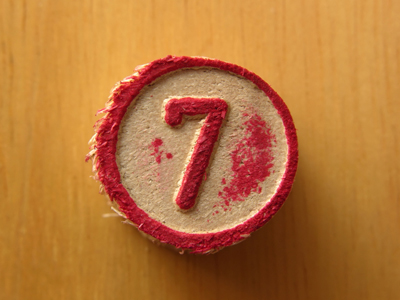7 is the digit in the hundreds' place in 3,701.

# Place Value (Very Easy)

Place values deal with tens, tenths and ones to name a few Look at these six numbers: 124, 142, 214, 241, 412, 421. They have been formed using only three digits, but they are all different because the digits have been put in different positions within the numbers: we say that the digits have different place positions and each place has a place value. In this 11-plus Maths quiz you will get the chance to revise place values in whole numbers < 10,000.

Use this information to help you find a digit's place value. Reading the number from right to left: the first digit is in the ones' place; the second digit is in the tens' place; the third digit is in the hundreds' place; the fourth digit is in the thousands' place.

For example, in 3,659 the 9 is in the ones' place; the 5 is in the tens' place; the 6 is in the hundreds' place; the 3 is in the thousands' place.

1.
What is the place value of 4 in 4,078?
The tens' place
The ones' place
The thousands' place
The hundreds' place
Reading the number from right to left: 4 is in the thousands' place
2.
What is the place value of 7 in 9,897?
The tens' place
The thousands' place
The hundreds' place
The ones' place
Reading the number from right to left: 7 is in the ones' place
3.
What is the digit in the hundreds' place in 3,701?
1
7
0
3
Reading the number from right to left: the 7 is in the hundreds' place
4.
What is the digit in the thousands' place in 4,501?
4
0
1
5
Reading the number from right to left: the 4 is in the thousands' place
5.
What is the digit in the tens' place in 4,029?
2
9
0
4
Reading the number from right to left: the 2 is in the tens' place
6.
What is the place value of 8 in 7,458?
The ones' place
The tens' place
The thousands' place
The hundreds' place
Reading the number from right to left: 8 is in the ones' place
7.
What is the place value of 5 in 3,507?
The ones' place
The thousands' place
The tens' place
The hundreds' place
Reading the number from right to left: 5 is in the hundreds' place
8.
What is the digit of the hundreds' place in 3,567?
5
6
7
3
Reading the number from right to left: the 5 is in the hundreds' place
9.
What is the digit in the ones' place in 5,709?
0
7
9
5
Reading the number from right to left: the 9 is in the ones' place
10.
What is the place value of 8 in 1,489?
The thousands' place
The ones' place
The hundreds' place
The tens' place
Reading the number from right to left: 8 is in the tens' place
Author:  Frank Evans